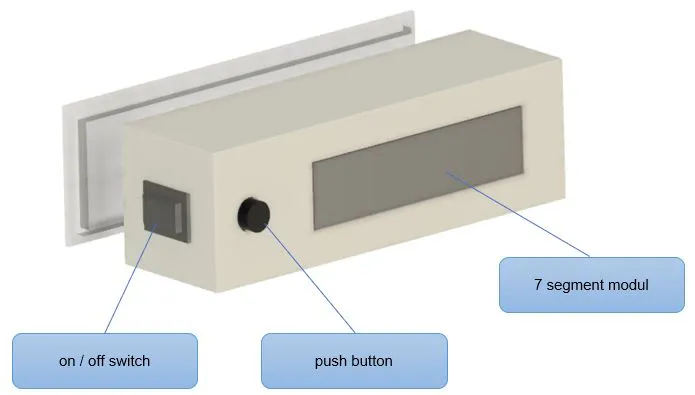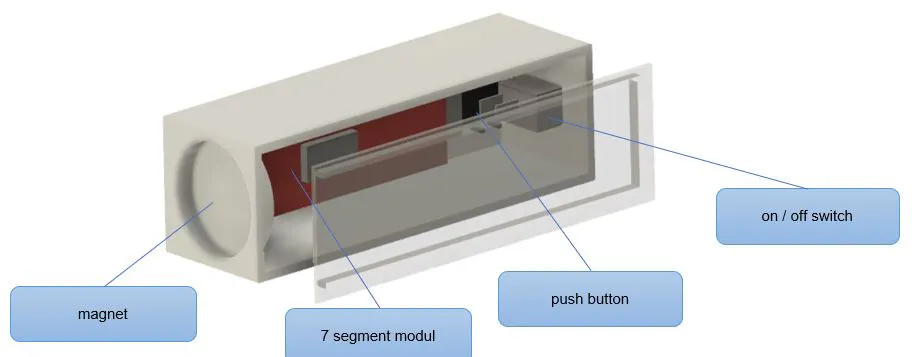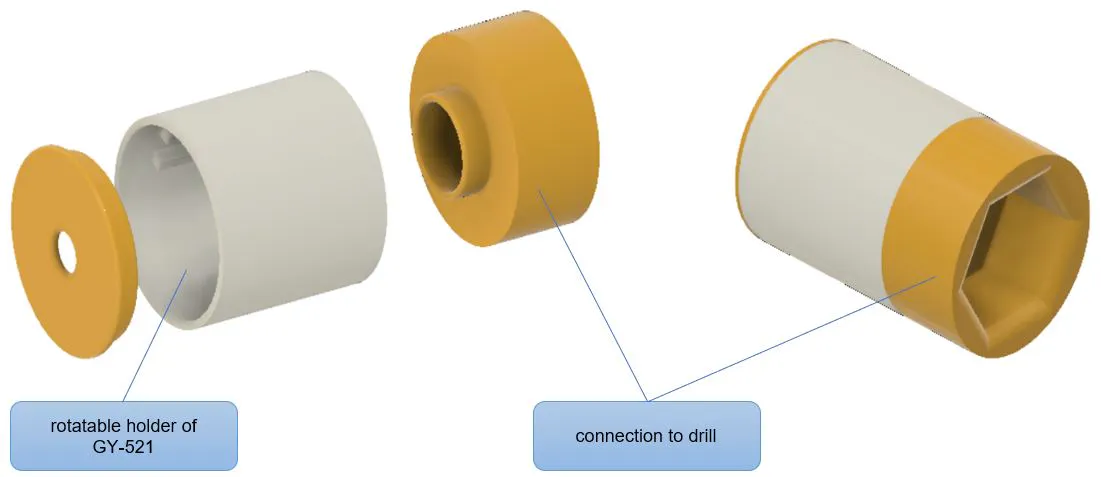• Arduino Nano
• 陀螺传感器GY-521
• 7位模块，带8位数字（SPI版本，带有MAX7219 IC）
• 按键
• 开/关
• 电源：AA或AAA电池的电池夹或移动电源
• 3D打印外壳
• 钕磁铁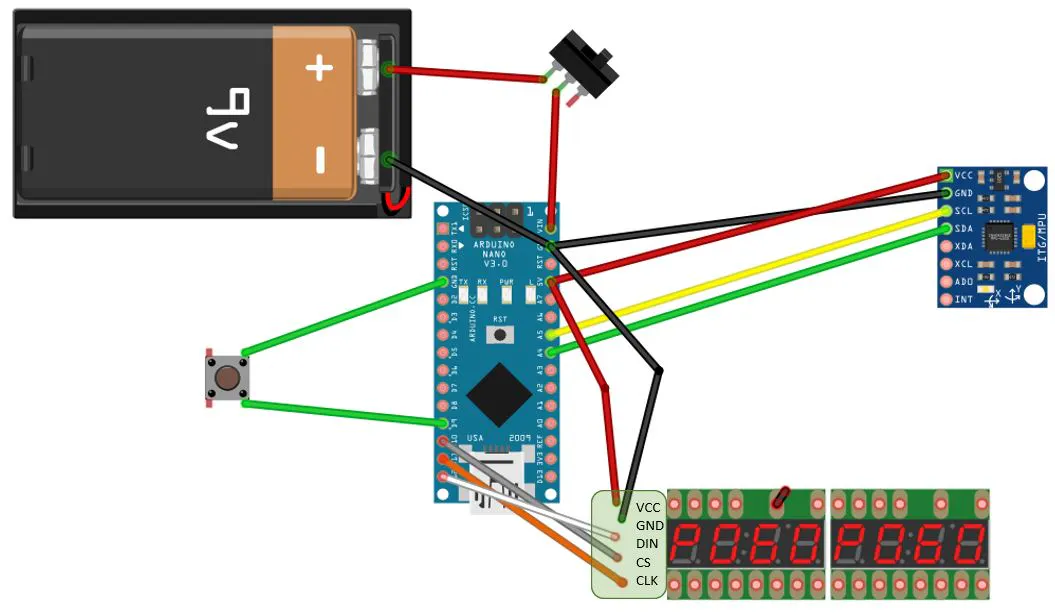1、陀螺仪传感器通过I2C连接。因此，对于Nano，必须使用A5和A4。

• VCC-> 5V
• 地线->地线
• SCL-> A5
• SDA-> A4
• XDA->未连接
• XCL->未连接
• ADO->未连接
• INT->未连接

2、7段模块：

• VCC-> 3.3V
• 地线->地线
• DIN-> D12
• CS-> D10
• 时钟-> D11

3、按钮：

4、电源供应：

5、套管：

/ *程序：rilling-depth.ino
*作者：托马斯·安吉尔斯基
*版本：2021 -03-20
*
* https://techpluscode.de/
* https://techpluscode.de/bohrtiefenanzeige-mit-gyro-sensor/
*
*此草图测量台式钻孔的钻孔深度
*使用GY-521陀螺仪传感器数据
*通过7段显示

*
*来自electronicoobs.com的calc误差补偿的想法-谢谢！
*
* /
#include <Wire.h>
#include <math.h>
#include "LedControl.h"

#define MPU 0x68

char txt;
String s;
float currentPos,stopPos;
float rad_to_deg;
float AccX, AccY, AccZ;
float AccAngleX, AccAngleY;
float AccAngleErrorX, AccAngleErrorY;
float TotalAngleX, TotalAngleY;
float DrillingMachineAngle, delta;

//初始化LED ：引脚D12，引脚D11，引脚D10、1个模块
LedControl lc=LedControl(12,11,10,1);
void setup() {
//准备串行连接
//Serial.begin(9600）;

//开始值
stopPos=0.0;
currentPos=0.0;
rad_to_deg = 180/PI;

//准备GY-521传感器
//我们仅使用加速器数据
Wire.begin();
Wire.beginTransmission(MPU);
Wire.write(0x6B);
Wire.write(0x00); // wake up MPU
Wire.endTransmission(true);

//delay（1000）
Wire.beginTransmission(MPU);
Wire.write(0x1C); //注册 ACCEL_CONFIG
Wire.write(0x10); //设置00010000 来实习 +/- 8g 满量程范围
Wire.endTransmission(true);

//计算加速器数据错误
//进行100次测量
for(int a=0; a<100; a++) {
Wire.beginTransmission(MPU);
Wire.write(0x3B);
Wire.endTransmission(false);
Wire.requestFrom(MPU,6,true);

AccX=(Wire.read()<<8|Wire.read())/4096.0 ;
AccY=(Wire.read()<<8|Wire.read())/4096.0 ;
AccZ=(Wire.read()<<8|Wire.read())/4096.0 ;

AccAngleErrorX=AccAngleErrorX+((atan((AccY)/sqrt(pow((AccX),2)+pow((AccZ),2)))*rad_to_deg));
//AccAngleErrorY=AccAngleErrorY+((atan(-1*(AccX)/sqrt(pow((AccY),2)+pow((AccZ),2)))*rad_to_deg));
}

AccAngleErrorX=AccAngleErrorX/100;
//AccAngleErrorY=AccAngleErrorY/100;

//准备按钮：D9针
pinMode(9,INPUT_PULLUP);

//准备7段显示
lc.shutdown(0,false);
lc.setIntensity(0,8);
lc.clearDisplay(0);
}

void loop() {
Wire.beginTransmission(MPU);
Wire.write(0x3B);
Wire.endTransmission(false);
Wire.requestFrom(MPU,6,true);

AccX=(Wire.read()<<8|Wire.read())/4096.0;
AccY=(Wire.read()<<8|Wire.read())/4096.0;
AccZ=(Wire.read()<<8|Wire.read())/4096.0;

AccAngleX=(atan((AccY)/sqrt(pow((AccX),2)+pow((AccZ),2)))*rad_to_deg)-AccAngleErrorX;
//AccAngleY=(atan(-1*(AccX)/sqrt(pow((AccY),2)+pow((AccZ),2)))*rad_to_deg)-AccAngleErrorY;

//平均值
TotalAngleX=0.5*(TotalAngleX)+0.5*AccAngleX;
//TotalAngleY=0.5*(TotalAngleY)+0.5*AccAngleY;

//使用X，Y，Z的+/-将x角度计算为360，增量= 0;

if ((AccZ<0)) {
delta=180.0-TotalAngleX*2.0;
}

if ((AccZ>0)&&(AccY<0)) {
delta=360.0;
}

DrillingMachineAngle=TotalAngleX+delta;
//如果接近360，则显示效果更好0
if (DrillingMachineAngle>350) {DrillingMachineAngle=0;}

//计算钻孔深度
//最大钻孔深度：50 mm（在机器上测量）
//手轮最大角度：316（使用Serial.print数据测量）
currentPos=50.0/316.0*DrillingMachineAngle;

/*
Serial.print("X / X / Y / Z / E : BOHRTIEFE");
Serial.print(TotalAngleX);
Serial.print(" ");
Serial.print(AccX);
Serial.print(" ");
Serial.print(AccY);
Serial.print(" ");
Serial.print(AccZ);
Serial.print(" >> ");
Serial.print(DrillingMachineAngle);
Serial.print(" >> ");
Serial.print(currentPos);
Serial.println(" ");
*/

//按下按钮？
if (digitalRead(9)==LOW) {
//储存停止位置
stopPos=currentPos;
lc.setChar(0,3,'8',false);
lc.setChar(0,2,'8',false);
lc.setChar(0,1,'8',false);
lc.setChar(0,0,'8',false);
//Serial.println("按下按钮");
delay(1000);
}

if (stopPos>0) {
//到达停止位置?
if (currentPos>=stopPos) {
//是：在右侧显示STOP
lc.setChar(0,3,'5',false);
lc.setRow(0,2,B00001111);
lc.setChar(0,1,'0',false);
lc.setChar(0,0,'P',false);
} else {
//否：显示到停止位置的距离
dtostrf(stopPos-currentPos,4,1,txt);
s=' '+String(txt);
lc.setChar(0,0,s.charAt(s.length()-1),false);
lc.setChar(0,1,s.charAt(s.length()-3),true);
lc.setChar(0,2,s.charAt(s.length()-4),false);
lc.setChar(0,3,s.charAt(s.length()-5),false);
}
} else {
//不显示任何内容
lc.setChar(0,3,' ',false);
lc.setChar(0,2,' ',false);
lc.setChar(0,1,' ',false);
lc.setChar(0,0,' ',false);
}

//在左侧显示当前钻孔深度
//格式化为字符串
dtostrf(currentPos,4,1,txt);
s=' '+String(txt);

lc.setChar(0,4,s.charAt(s.length()-1),false);
lc.setChar(0,5,s.charAt(s.length()-3),true);
lc.setChar(0,6,s.charAt(s.length()-4),false);
lc.setChar(0,7,s.charAt(s.length()-5),false);

delay(200);
}

1、使用LedControl.h库来控制7段显示。如未安装，则必须使用库管理器进行安装。

2、陀螺传感器通过I2C总线连接。通过Wire.h进行通信。

3、使用Led Control控制7段显示。

4、引脚进行初始化后，设置过程仅需要进行一些准备工作即可唤醒显示并调整强度。

5、在循环部分中，显示屏仅显示当前钻孔深度，如果已设置，则显示停止位置，显示为数字。

6、函数dtostrf将浮点数转换为字符数组。然后输入一个字符串，以在显示屏上按字符显示它。8、通过线库的功能读取陀螺传感器的功能。使用加速度计来确定角度位置。

9、当USB连接到Arduino IDE时，一切运行正常。拔下插头并连接到外部电源后，传感器未提供任何数据。它仅在Arduino复位后才能再次工作。经过长时间的搜索，解决方案是在唤醒传感器后安装等待时间。这使延迟（1000）在整个源代码中成为最重要的命令。X角度（度）使用以下公式计算：

AccAngleX = (atan ((AccY) / sqrt (pow ((AccX), 2) + pow ((AccZ), 2))) * rad_to_deg);

TotalAngleX = 0.5 * TotalAngleX + 0.5 * AccAngleX;currentPos=50.0/316.0*DrillingMachineAngle;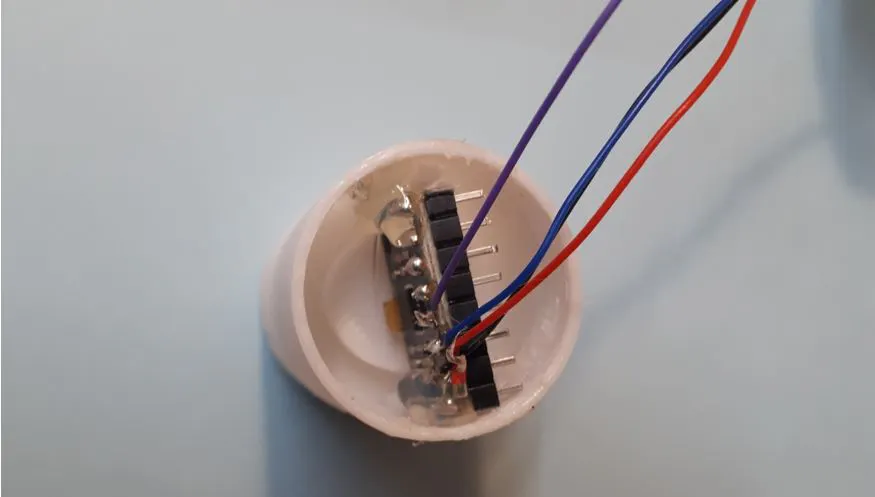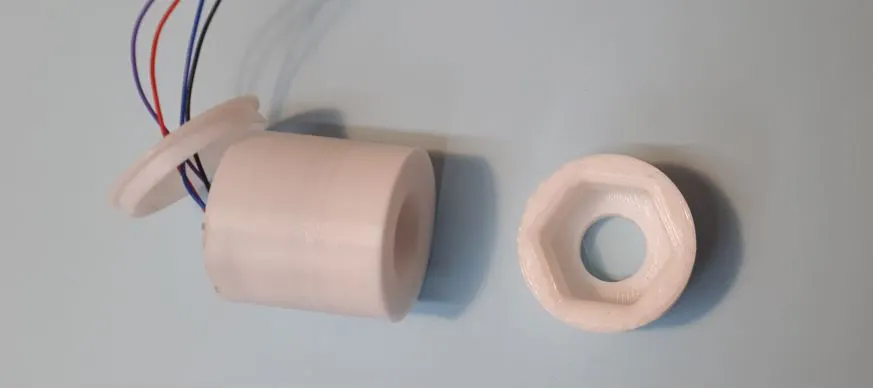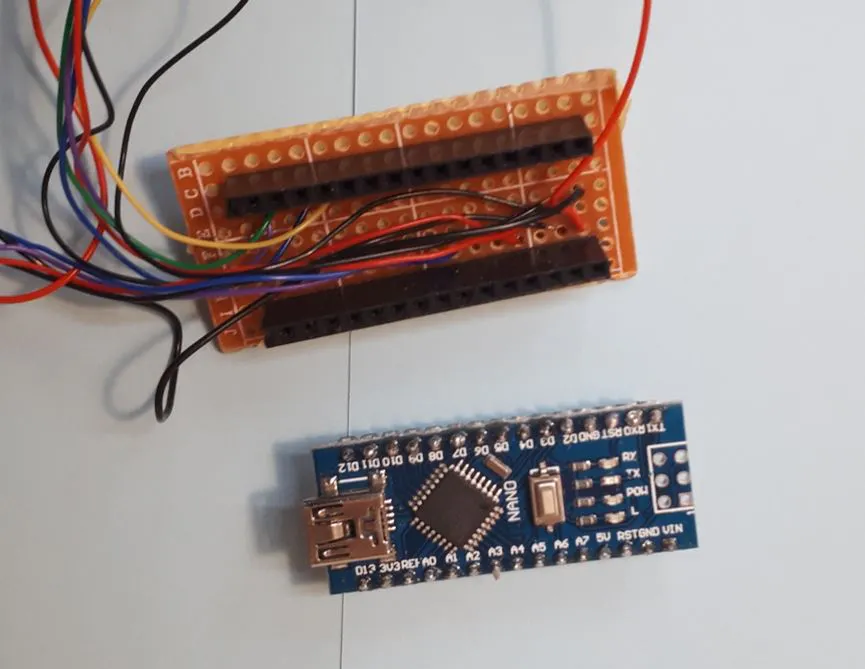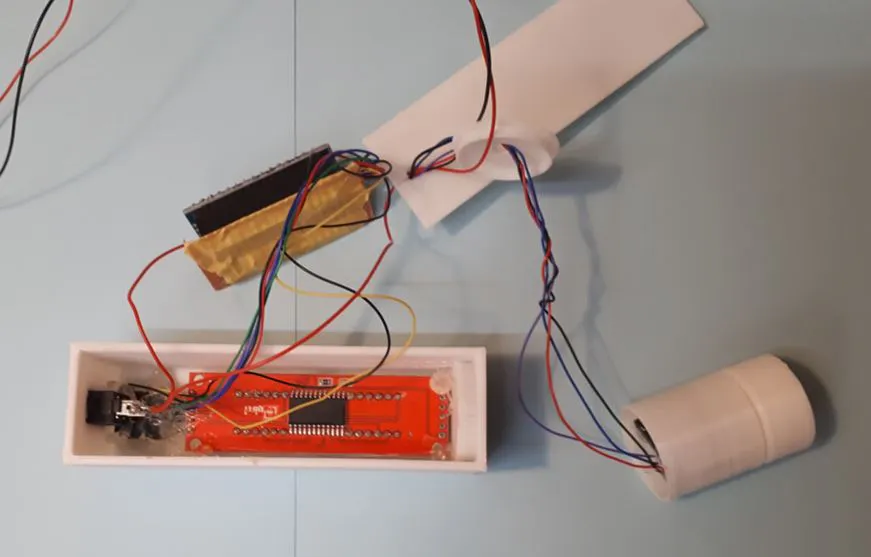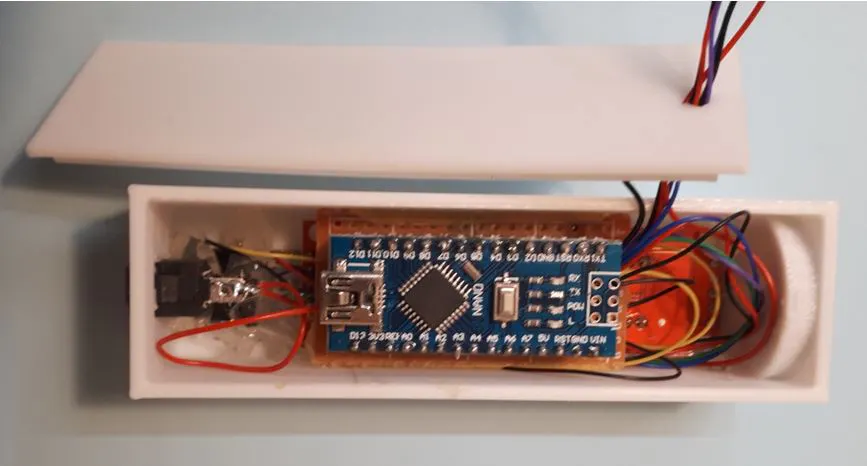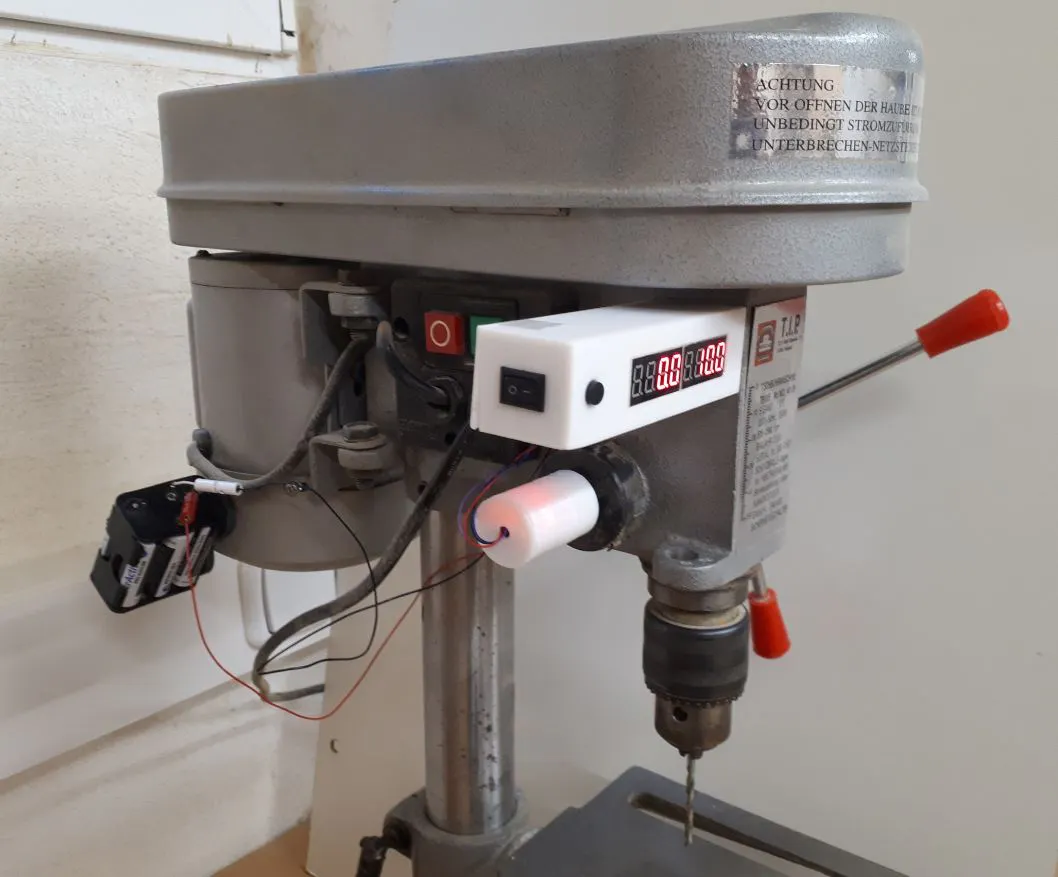加入微信技术交流群关注与非网服务号加入电路城 QQ 交流群